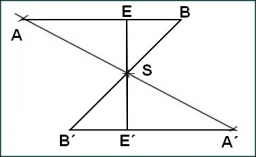# Triangle 35881

The sum of the lengths of the two sides b + c = 12 cm
Beta angle = 68
Gamma angle = 42
draw triangle ABC

a =  7.064 cm
b =  6.9699 cm
c =  5.0301 cm

### Step-by-step explanation:

Try calculation via our triangle calculator.Did you find an error or inaccuracy? Feel free to write us. Thank you!

Tips for related online calculators
Do you want to convert length units?

#### Grade of the word problem:

We encourage you to watch this tutorial video on this math problem: